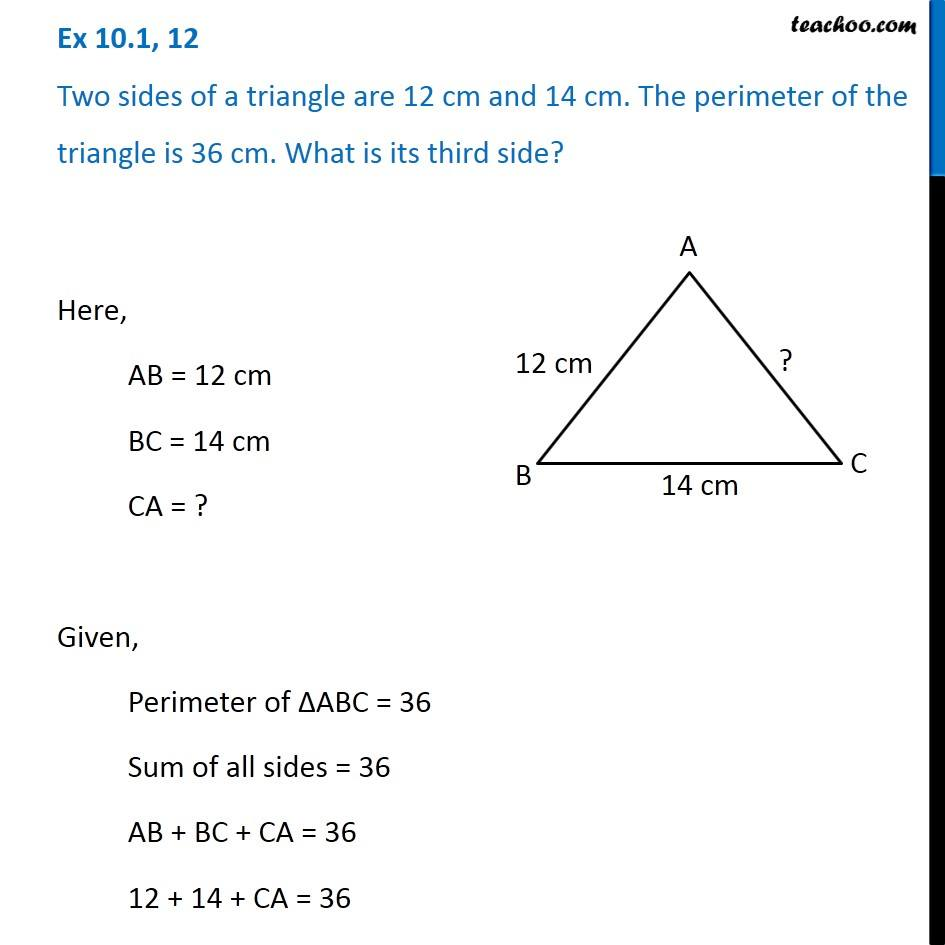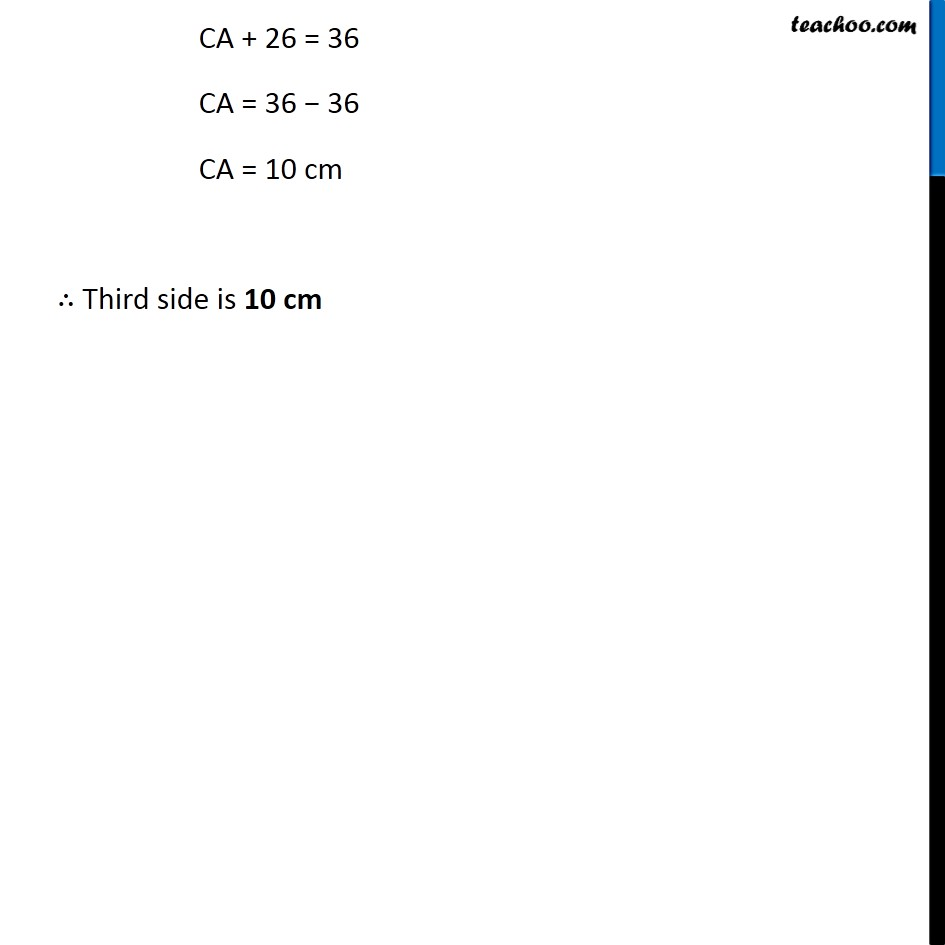Ex 10.1

Chapter 10 Class 6 Mensuration
Serial order wiseLearn in your speed, with individual attention - Teachoo Maths 1-on-1 Class

### Transcript

Ex 10.1, 12 Two sides of a triangle are 12 cm and 14 cm. The perimeter of the triangle is 36 cm. What is its third side? Here, AB = 12 cm BC = 14 cm CA = ? Given, Perimeter of ∆ABC = 36 Sum of all sides = 36 AB + BC + CA = 36 12 + 14 + CA = 36 CA + 26 = 36 CA = 36 − 36 CA = 10 cm ∴ Third side is 10 cm